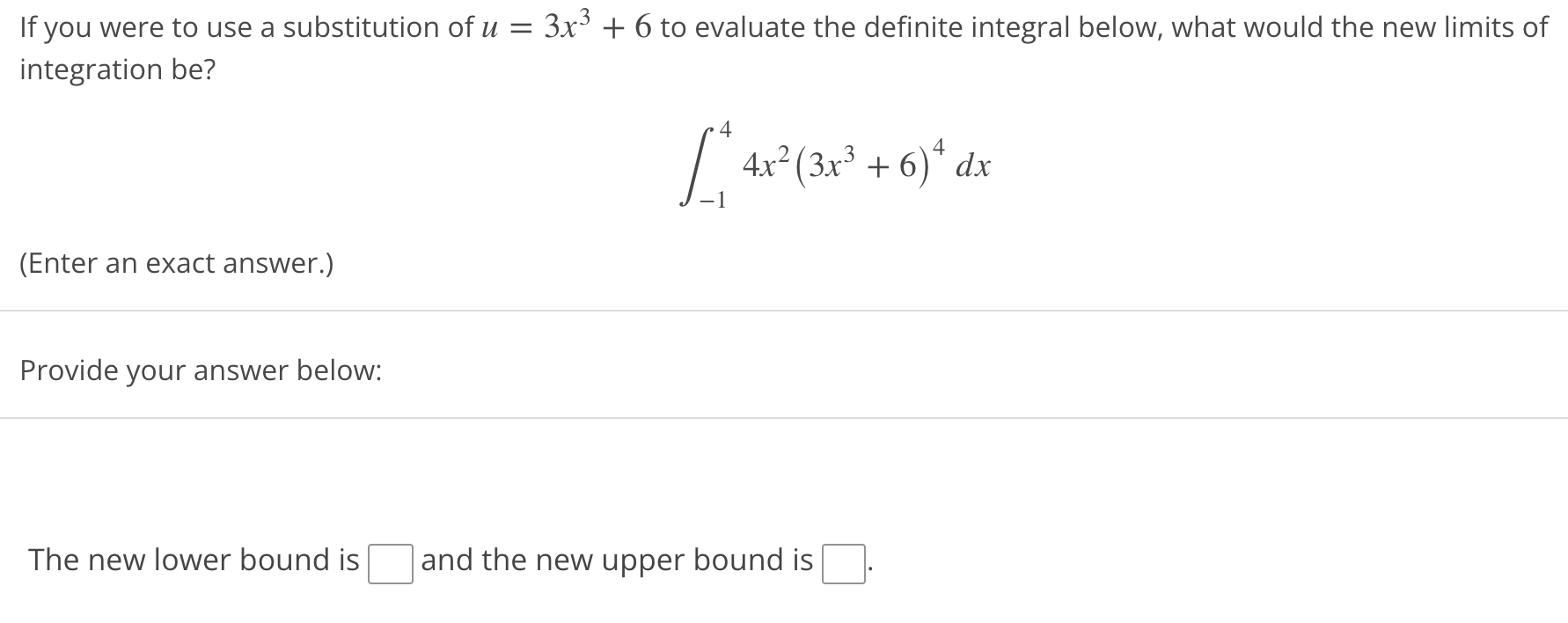# Question Solved1 Answer= If you were to use a substitution of u = 3x3 + 6 to evaluate the definite integral below, what would the new limits of integration be? 4 4 [ 4x2 (3x3 + 6)* dx +6; (Enter an exact answer.) Provide your answer below: The new lower bound is and the new upper bound isTranscribed Image Text: = If you were to use a substitution of u = 3x3 + 6 to evaluate the definite integral below, what would the new limits of integration be? 4 4 [ 4x2 (3x3 + 6)* dx +6; (Enter an exact answer.) Provide your answer below: The new lower bound is and the new upper bound is
More
Transcribed Image Text: = If you were to use a substitution of u = 3x3 + 6 to evaluate the definite integral below, what would the new limits of integration be? 4 4 [ 4x2 (3x3 + 6)* dx +6; (Enter an exact answer.) Provide your answer below: The new lower bound is and the new upper bound is